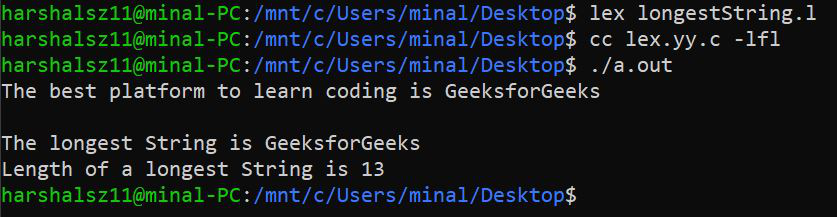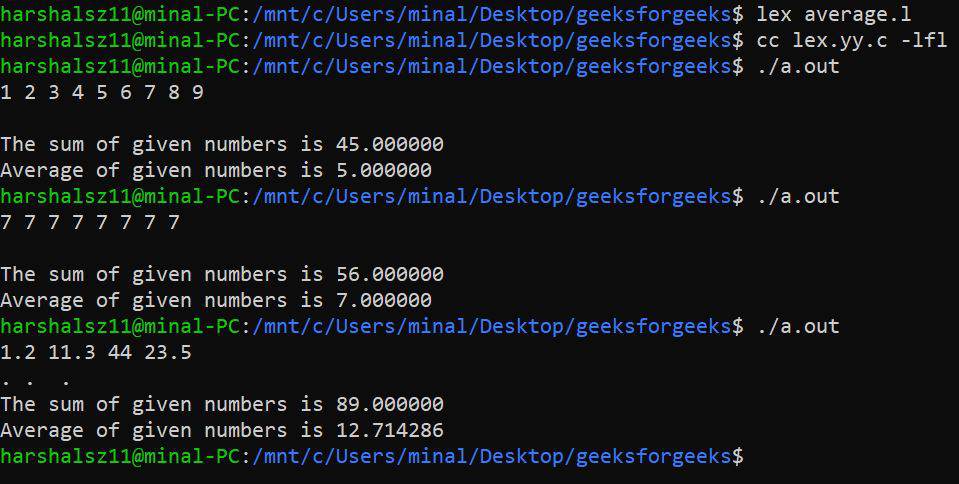# LEX program to print the longest string and to find average of given numbers

• Last Updated : 03 Aug, 2021

Lex :
The Lex program has purpose of generating lexical analyzer. Lex analyzers are a program that transform input streams into sequence of tokens. A C program implements the lexical analyzer to read input stream and produce output as the source code.

Commands to be used –

```lex file_name.l   // To produce a c program
cc lex.yy.c -lfl  // To compile the c program and produces object program a.out.
./a.out           // To transforms an input stream into a sequence of tokens.    ```

1. Longest string :
In this we are finding the length of longest string by using the function called( It returns the length of a given string). And we are storing this returned value in the variable. To print what string or word is longest we are usingandfunction. We are storing the string or word in a variable of type char that is.

Program –

## C

 `%{ ` `   ``//to find longest string and its length ` `    ``#include ` `    ``#include ` `    ``int` `longest = 0; ` `    ``char` `longestString; ` `%} ` `%% ` `[a-zA-Z]+ { ` `if``(yyleng>longest){ ` `    ``longest = yyleng; ` `    ``strcpy``(longestString,yytext); ` `} ` `} ` ` `  `%% ` ` `  `int` `main(``void``){ ` `    ``yylex(); ` `    ``printf``(``"The longest string is %s \n"``, longestString); ` `    ``printf``(``"Length of a longest string is %d \n"``,longest); ` `} `

Output –2. Average of given numbers :
So, first we have to calculate sum of all numbers that are given by user. Store sum in a variable sum. Then count number of integers(numbers that are given by the user). Store this count in a variable n.
After this, just divide the sum and count of numbers. You will get your result.
Here, we are using inbuild function atoi(). A string of characters can be passed into atoi() function, which will convert them into an integer. The input string is a text string that can be converted into a numeric value. When first character in input string is not part of a number, atoi() function stops reading it. This could be null character at the end of string. It does not support exponents and decimals.
The atoi() function removes all the Whitespace in string at beginning.

Program –

## C

 `%{ ` `    ``//Average of given numbers. ` `    ``#include ` `    ``#include ` `%} ` `digit[0-9]+ ` `%% ` `{digit} ``return` `atoi``(yytext); ` `%% ` ` `  `int` `main(){ ` `    ``float` `sum=0, num, n=0; ` `    ``while``((num=yylex())>0){ ` `    ``sum = sum+num; ` `    ``n = n+1; ` `} ` `printf``(``"The sum of given numbers is %f\n"``,sum); ` `printf``(``"Average of given numbers is %f\n"``,sum/n); ` `yylex(); ` `return` `0; ` `} `

Output –My Personal Notes arrow_drop_up
Recommended Articles
Page :